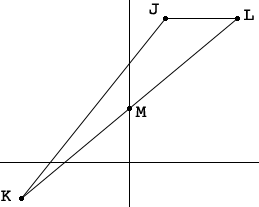SEARCH HOMEMath Central Quandaries & QueriesQuestion from Anna: For triangle J(1,4) K(-3,-1) L(3,4), find an equation for the median from vertex JHi Anna,

I would draw a diagram. I always draw a diagram.The median from $J$ is the line that goes through $J$ and the midpoint of the line segment $KL.$ On my diagram, $M$ is this midpoint. I assume you can writ the equation of a line through two points if you know their coordinates, hence I need the coordinates of $M.$

Since $M$ is the midpoint of the line segment $KL$ the x-coordinate of $M$ is half way between the x-coordinates of $K$ and $L.$ This midpoint is $\large \frac{-3 + 3}{2} \normalsize = 0.$

What is the y-coordinate of $M?$ What is the equation of the line through $K$ and $L.$

PennyMath Central is supported by the University of Regina and The Pacific Institute for the Mathematical Sciences.